# Team:HSiTAIWAN/SoftwareX

Oh hello there,I am Charlie,a trusty member of the "Herb Tasters" and also the brainiest E. coli in the colony.
I know all the secrets of Chinese herbs and their magical healing powers.
If you are up to a challenge,find me at team HSiTW at the jamboree.
I am the one in a straw hat,showing them pearls.I will be waiting.
Muhahahahaha

Hi there! My name is Nu Zhen Chi. This is how I look like.
Take a closer look; guess which part of me is used as medicine?
(1) the root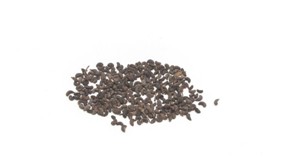(2) the stem
(3) the leaf
(4) the seed

Ans.(4) the seed
Name: 女貞子 (Nu Zhen Chi)
Botanical Name: Ligustrum lucidum Aiton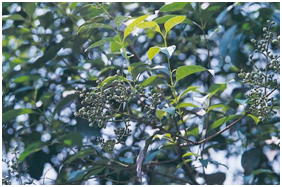I can treat people who are yin deficient, and liver problems that cause dizziness,cataract of the eyes,
lower back pain, premature graying of the hair and tinnitus.

Hello! My name is Chuan Xiong. This is how I look like.
Make a guess, which part of me is used as medicine?
(1) the root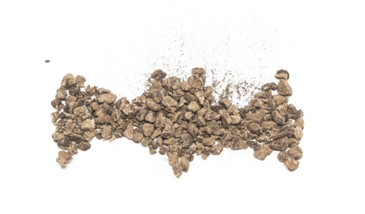(2) the stem
(3) the leaf
(4) the seed

Ans.(1) the root
Name: 川芎 (Chuan Xiong)
Botanical Name: Ligusticum chuanxiong Hort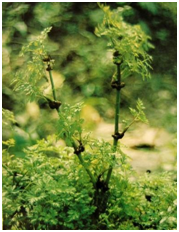I help with blood regulation to prevent relevant to blood stasis and non-stop bleeding.I can also strengthen your qi circulation.
In addition, I relieve you of physical pain, such as headaches, abdominal aches, chest pain, and muscle pain.
Finally, I free the ladies of menstrual disorders and amenorrhea.

What’s up? My name is Dang Gui. I can:
(1) stop coughing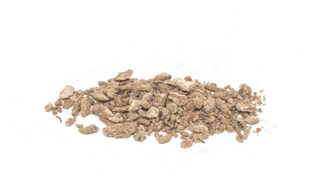(2) regulate mense
(3) reduce internal heat

Ans.(2) Regulate mense
Name: 當歸 (Dang Gui)
Botanical Name: Angelica sinensis (Oliv.) Diels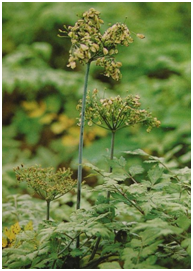I can remove blood stasis and clots, so I am usually used to regulate menses,lubricate intestines to correct constipation, reduce swelling, expel pus.

Reference
臧堃堂 (2005) 中華材輕百科-現代版本草綱目，山岳文化出版社，台北
Non-Profit Organization Brion Research Institute of Taiwan.
Herbal Glossary. Shen-Nong- Chinese Traditional Medicine
Acknowledgement
Thank you for Non-Profit Organization Brion Resaerch Institute of Taiwan that provide us Chinese herbs and photos.

Next ⇒
⇐ Prev
...

• Binding K

• Introduction
• Calculate Binding Energy
• Simulation
• Software
• Reference
•

Binding K

The phase space related to various parameters plays an important role for scientists in the bio-engineering field. The appropriate simulation of the genetic circuits by software will be helpful for design of genetic switch. Based on the synthetic genetic circuits with non-coding small RNA, we study the phase space of binding constant K (Kb) for engineering in Escherichia coli (E. coli). The various types of sRNA mediated circuits are well described by relevant Kb, mapping the near simultaneously regulations to decrease (or increase) the target mRNA. The popular genetic switch, Plac with LacI (weak binding) and Ptet and TetR (strong binding), are used here to mimic the simplest synthetic circuits in E. coli. We develop the software tool, Binding K, to illustrate how the genetic regulation varies with Kb. In addition, the mean field theory and the 4th Runge-Kutta method are integrated in this software tool.

The introduction of Binding K

The calculation of binding constant K for genetic circuits based on the non-coding RNA (sRNA) plays an important role for various types of bio-function. Even for E. coli, there are more than fifty types of sRNA mediated bio-function. To tackle the problem of the binding constant K for sRNA duplex in E. coli, we use some methods for the calculations. Our team member Eddie Wang, cooperating with Mr. Cheng-Ping Jheng, professor Cheng-I Lee (Department of Life Science, National Chung Cheng University, Taiwan) and instructor P. H. Lee have published one MD method to measure the binding constant K . Firstly, we set up the build-up of sRNA structure in E. coli. Secondly, the molecular dynamic (MD) and Amber molecular dynamic package calculating the A-form RNA duplex in implicit solvent model are used here. With the explicit solvent model, we take the Molecular Mechanics/Poisson Boltzmann Surface Area (MM/PBSA) as the basic modeling to calculate the change of Gibbs free energy in the formation of an RNA duplex. Finally, based on the calculation of Gibbs free energy, we fit the unknown binding K for the database of well-known sRNA, like RyhB and sodB, and then integrate this complex into the simulation code to predict the phase space for the sRNA mediated genetic circuits.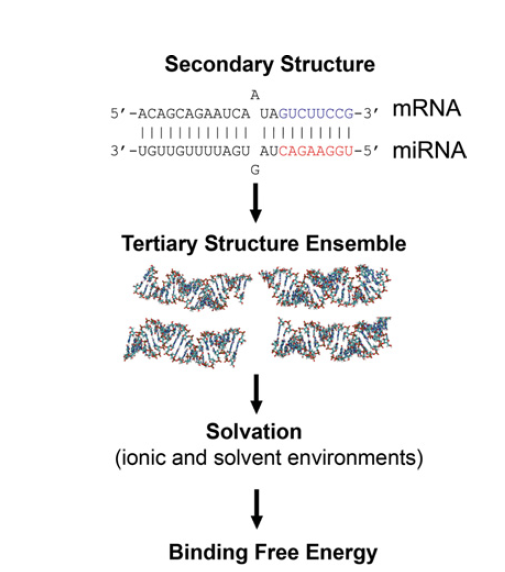Figure 1. The flow to calculate the binding energy

Calculate binding energy

The software list:

Amber14, AmberTools 15, Matlab, VMD

Community ENTerprise Operating System 6.7 with Intel parallel studio XE 2015 update 6

NVIDIA CUDA Toolkit version 5.0

The hardware list:

Equipped with quad-core and eight thread Intel Xeon E5 1620V2 processors with two GPUs of NVIDIA GeForce GTX Titan Black.

The usage for Binding K

Step 1: Find the binding site for small RNA and the related target mRNA in the BioCyc website. (BioCyc)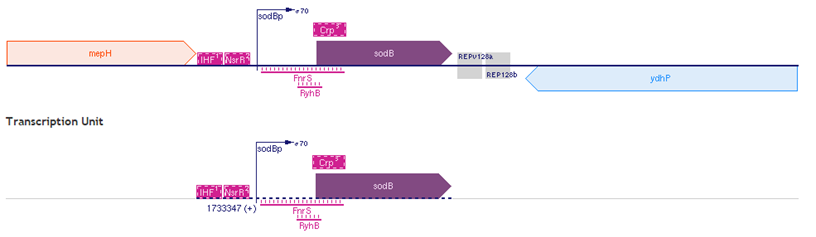Figure 2. The sodB DNA sequences.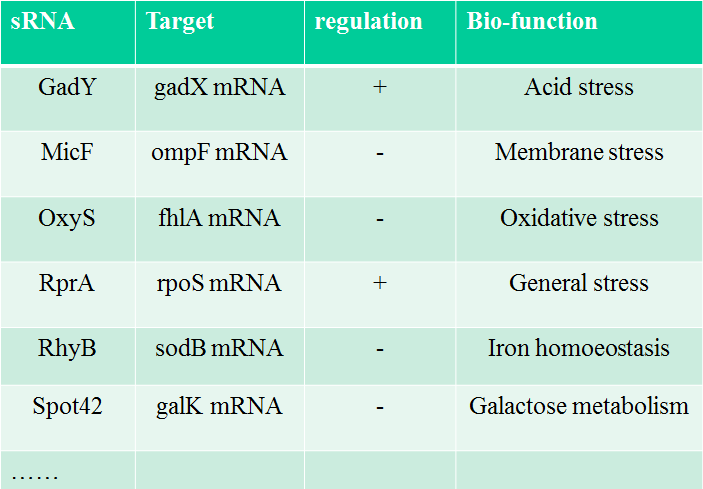Table 1. The famous sRNA in E. coli and their bio-function.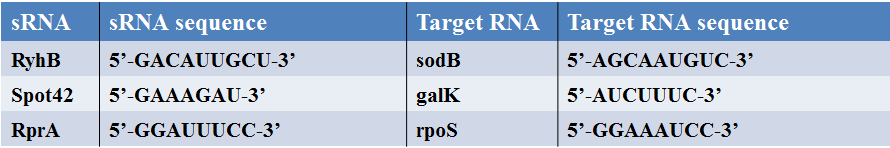Table 2. The sequences for 3 kinds of small RNAs and related target mRNAs.

Step 2: Use ncbi blastn to find the binding part. (ncbi blast)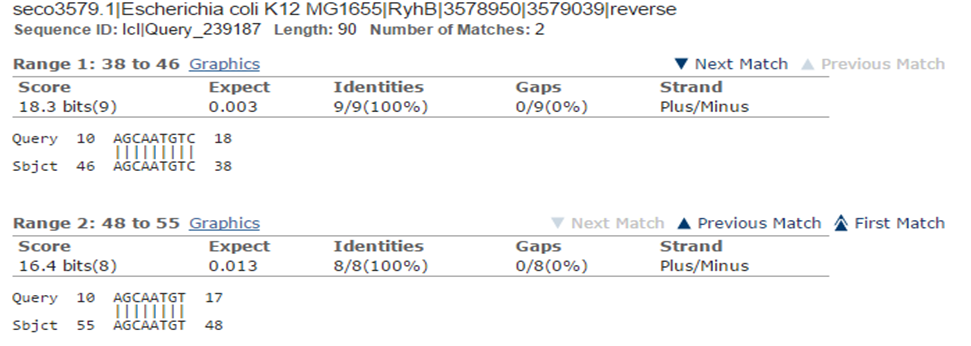Figure 3. Use ncbi blast to find the related target mRNAs.

Step 3: Use the Make-na server to generate the A-form RNA. (Make-na)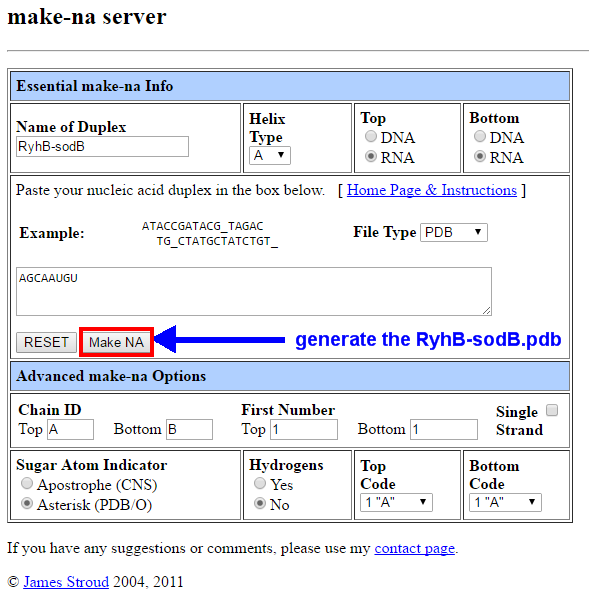Figure 4. Use Make-na server to generate the RyhB-sodB.pdb file.

Step 4: Use the H++ server to generate the amber input file. (H ++)Figure 5. Generate PDB (PQR) structure, Amber (implicit solvent) Coordinate File, Amber (explicit solvent) Topology File and Amber (explicit solvent) Coordinate File.

Step 5: Run the Amber code to calculate the structure with the implicit model. (Amber)

---------Basic Steps for Running Simulation-------

(1) Obtain starting Coordinates (PDB, NMR, Database, Program generated)

(2) Run LEaP to generate the parameter and topology file.

(3) Run Simulation (sander or pmemd)

(4) Analyze the results (ptraj)

We provide some AMBER setting here.

Script 1 (Linux)

----------min.in----------------
RyhB-sodB: initial minimization prior to MD GB model
&cntrl
min   = 1,
maxcyc = 500,
ncyc   = 250,
ntb    = 0,
igb    = 1,
cut    = 999
/


Script 2 (Linux)

----run-mini-------------
\$AMBERHOME/bin/pmemd.cuda -O -i min.in -o min.out -c RprA-rpoS.crd -p RprA-rpoS.top -r min.rst


Script 3 (Linux)

-------------converge RNA structure to the lower energy level-------------
Input: min.in run-min
>>nohup sh run-min &
Output: min.out min.rst
Form pdb: >>ambpdb –p RyhB-sodB.top  min.pdb
Open min.pdb by VMD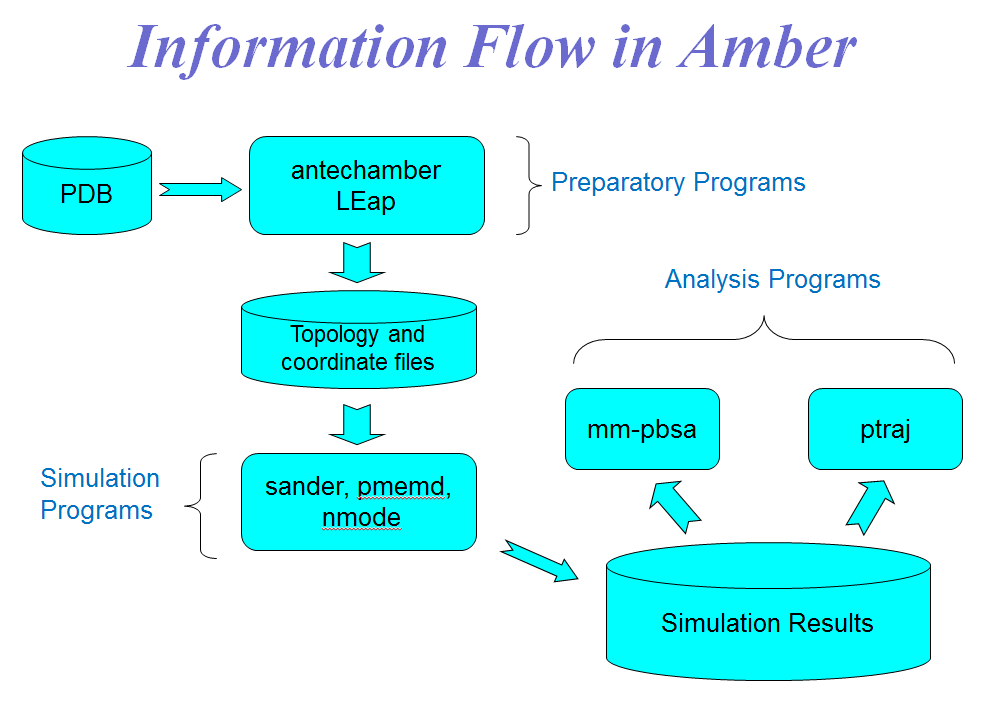Figure 6(a) Information Flow in Amber.Figure 6(b) Preparation of control data for the minimization/MD run.

Step 6: Run the Amber code to calculate the binding energy of complex structure with explicit model. (MMPBSA)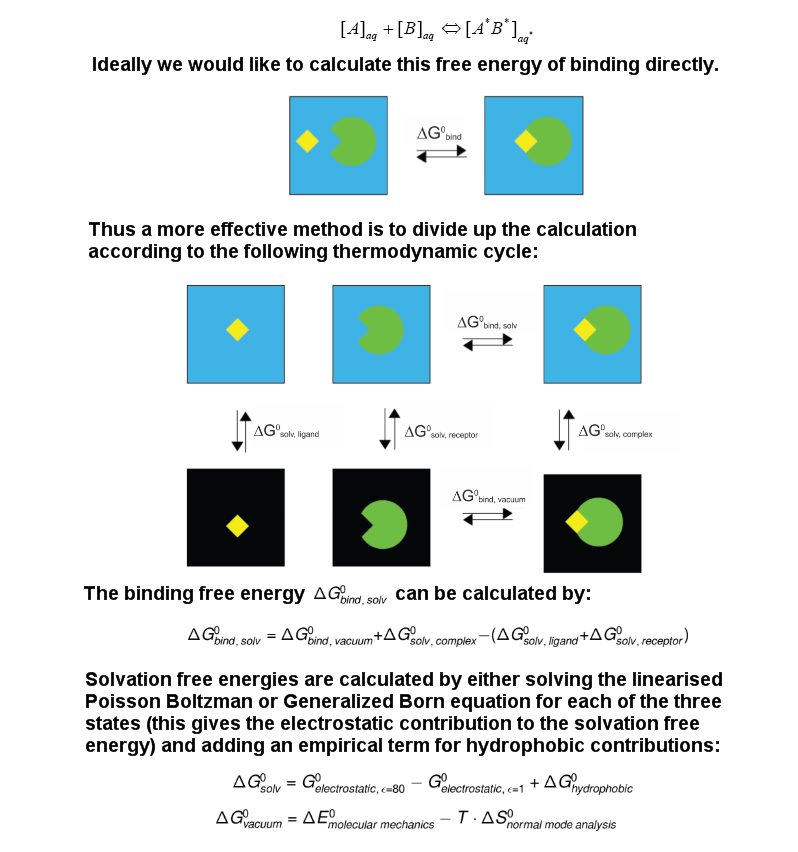Figure 7. The overall objective of the MM-PBSA method is to calculate the free energy difference between two states which most often represent the bounded and unbounded state of two solvated molecules or alternatively to compare the free energy of two different solvated conformations of the same molecule.

The simulation of sRNA complex

We simulate the sRNA-target complex in implicit solvent model based on AMBER molecular dynamic package. The all-atom molecular simulations are run by using force field ff14SB, the standard pairwise Generalized-Born solvation model (igb =1) and calculated with GPU-accelerated PMEMD.cuda. The RNA simulation in implicit solvent model is run by using the 500 steps of minimization and 820 ns at 300K. The unbounded cutoff is set to 999 Å in the minimization. For RMSD of steady states of RNA structure, we use the cpptraj program in AmberTools packages to analyze. The final structures of RNA duplex are converted into pdb files for the next step of MM/PBSA.

The MM/PB(GB)SA in explicit model

Following the previous step, the structures of RNA duplexes are divided into two parts in single strand RNA. The force field is ff14SB and the TIP3P water extends to 12 Å in a rectangular box with neutralized charges of RNA with sodium ions. The Radii parameter for non-polar solvation is mbondi in . Before MD, the solvated complex was relaxed by 1000 steps for minimization, 50 ps for heating from 0K to 300K, 50 ps for density equilibrium at 300K, and 500 ps for constant pressure equilibrium. In the MD step, total time is 2 ns in RNA simulation. The MM minimization and MD simulations are run by using the pmemd.cuda program in AMBER 14. The unbounded cutoff of 999 Å is also used in the minimization process. Finally, we use the scripts of MMPBSA.pl, one of AmberTools, to calculate the binding energy of sRNA-target duplex.

According to Figures 8-10 shown as RyhB-sodB, Spot42-galK, and RprA-rpoS, we calculate the structure of sRNA-target RNA in the implicit solvent model first.(Generalized-Born solvation model, GB). After MD step, the large changes of molecular positions occur in the final structures of sRNA-target RNA complex. Moreover, both of the distortion in the two ends of RNA structure and the bending of RNA backbones also affect the binding energy. Finally, we will compare these pictures with the method of MMPBSA.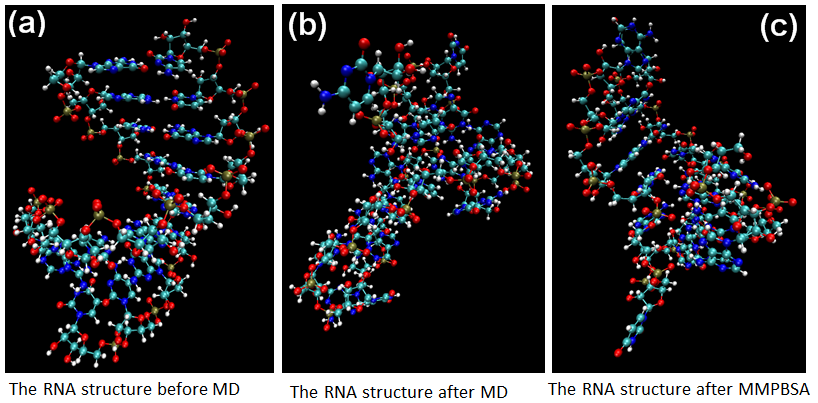Figure 8. The MD calculation for sRNA-mediated binding of RyhB and sodB.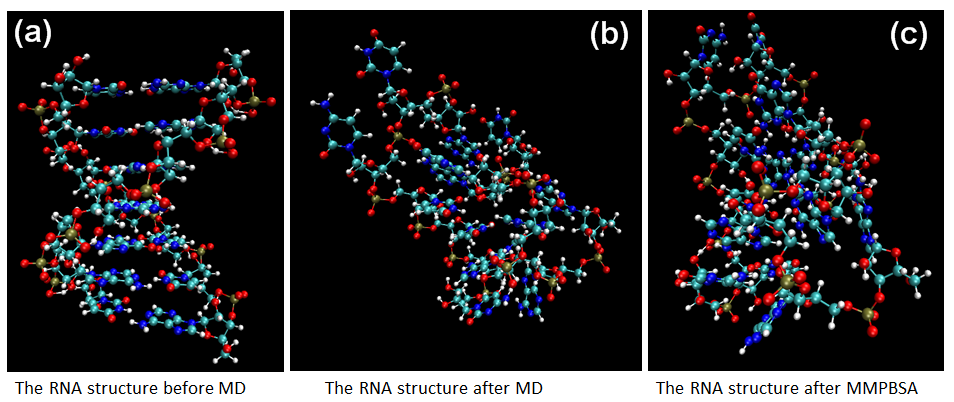Figure 9. The MD calculation for sRNA-mediated binding of Spot42 and galK.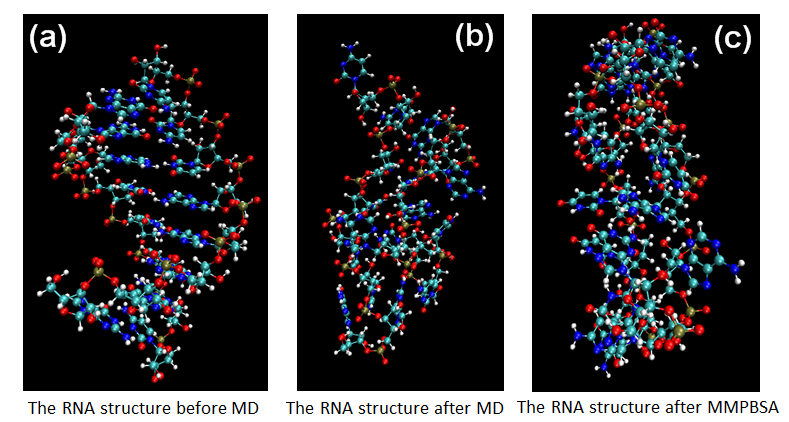Figure 10. The MD calculation for sRNA-mediated binding of RprA and rpoS.Figure 11. For RyhB-sodB, the density, temperature, total energy and backbone RMSD in the equilibrium state of the solvated complex based on the MM/PBSA calculation. (a) Density (b) Temperature (c) Total energy (d) Backbone RMSD.Figure 12. For RyhB-sodB, the scaled pictures of density, temperature, total energy and backbone RMSD in the equilibrium state of the solvated complex based on the MM/PBSA calculation. (a) Density (b) Temperature (c) Total energy (d) Backbone RMSD.

Software: The MM/PBSA calculation and coding for parameters

Considering the Equation 1 and Fig. 13, we try to map the difference of Gibbs free energy to the binding constant K for approximation approach. Due to the fact that the relationship of delta Gon and difference of Gibbs free energy is hard to investigate, we use the MM/PBSA method to mimic the binding constant K. It is intuitive that higher difference of Gibbs free energy and the stronger the binding constant.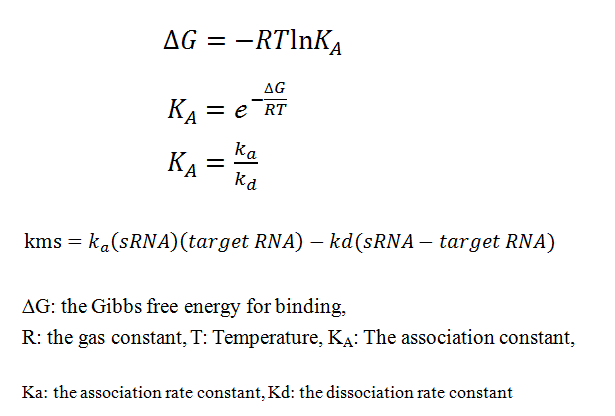Equation 1. The relationship of Gibbs free energy and the association constant KA.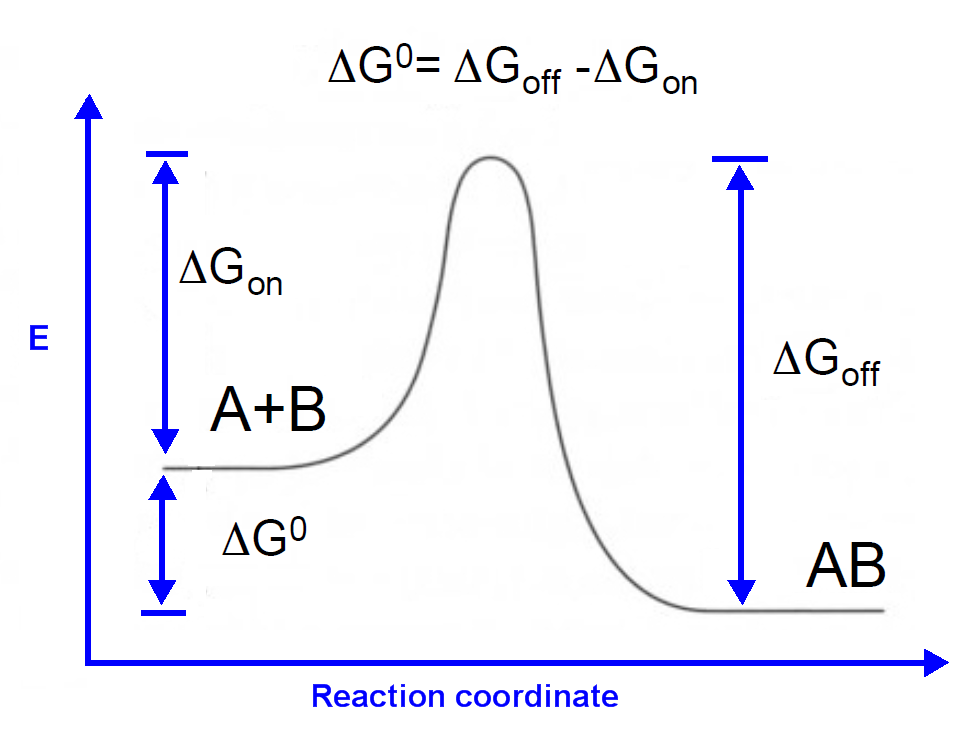Figure 13. The Gibbs free energy for sRNA-mediated binding.

The calculations with PBSA are listed in the Table 3. The RNA or DNA structures are often stabilized by the hydrogen bond (H-bond). This H-bond, however, does not affect the binding energy of RNA duplex a lot in our study, meaning that there is no obvious fact of lower Gibbs free energy with higher CG. The distortion of structure is the main reason to affect the contribution of H-bond in RNA binding. According to this issue, the variations of MD calculations exist in the absolute binding energy . For correction this, we analyze the fitting for the experimental binding energy vs. calculated energy in PBSA model. Some important papers for describing MM/PBSA methods indicate that the usage of PBSA are more reliable [16, 17].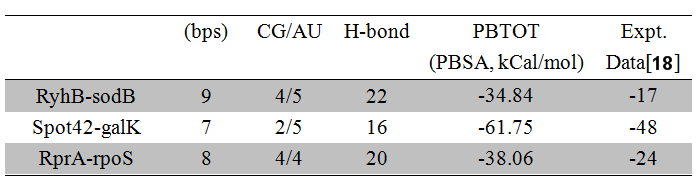Table 3. The MD calculation for Gibbs Free energy for sRNA-mediated binding.

We illustrate the good-fitting in Fig. 14 for the relation of experimental energy vs. MD calculated energy. This result implies the existence of linearity relationship among the binding energies of experimental and MD calculated. Therefore, it is possible to use this result to mapping the binding constant K from RyhB-sodB to the others sRNA-target.Figure 14. The correlation of MD calculated energy and experimental energy for various sRNA-mRNA.

To connect to the binding constant K, we design the synthetic regulatory circuit in Escherichia Coli based on the switch of RyhB-sodB as shown in Fig. 15. The mean-field theory is utilized in describing the rate equation 2-4. With the Runge-Kutta 4 order, the analyses of the phase space for Fig.15 are illustrated in Fig. 16 (K=0.02) and 17 (K=0.002).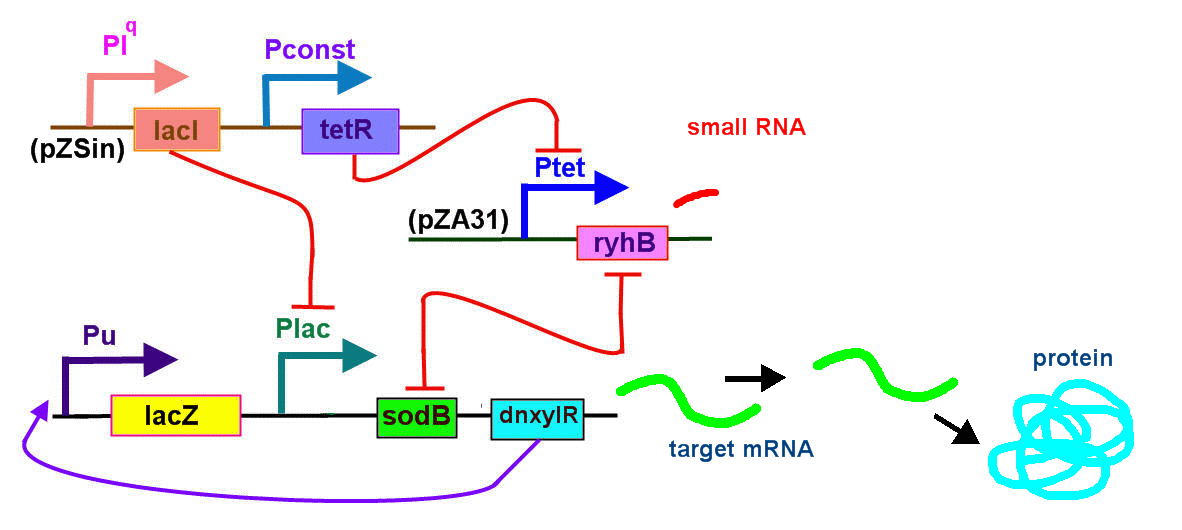Figure 15.The sRNA-mediated regulation circuit and the rate equations. Here the reporter is LacZ or CYP.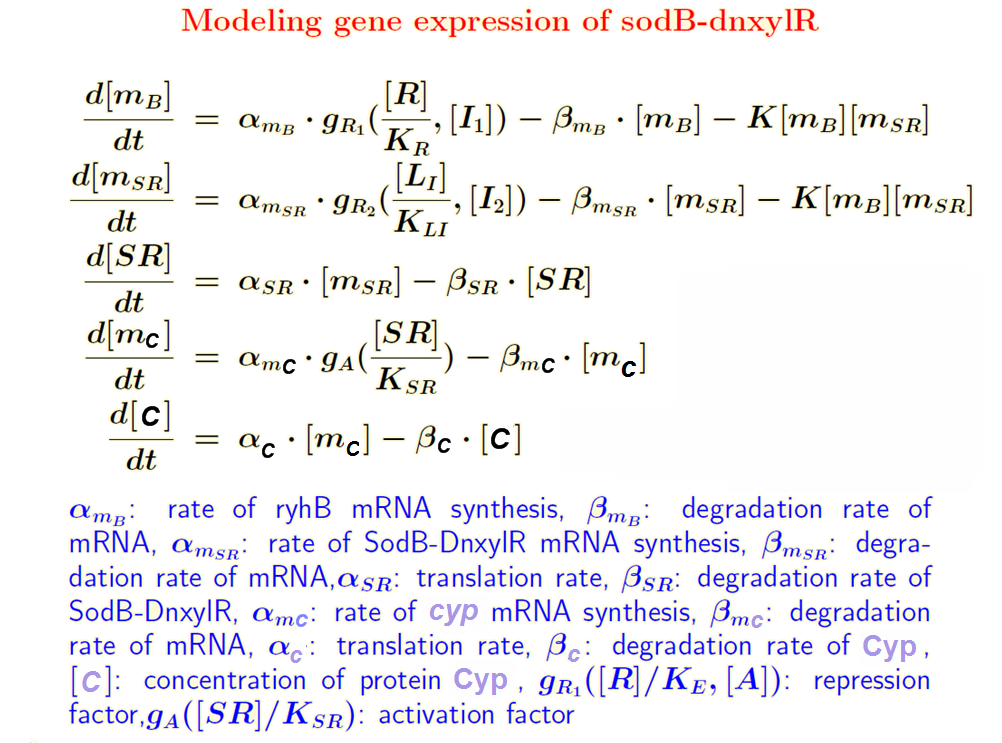Equation 2: The mean field theory is used to describe the sRNA-mediated regulation circuit.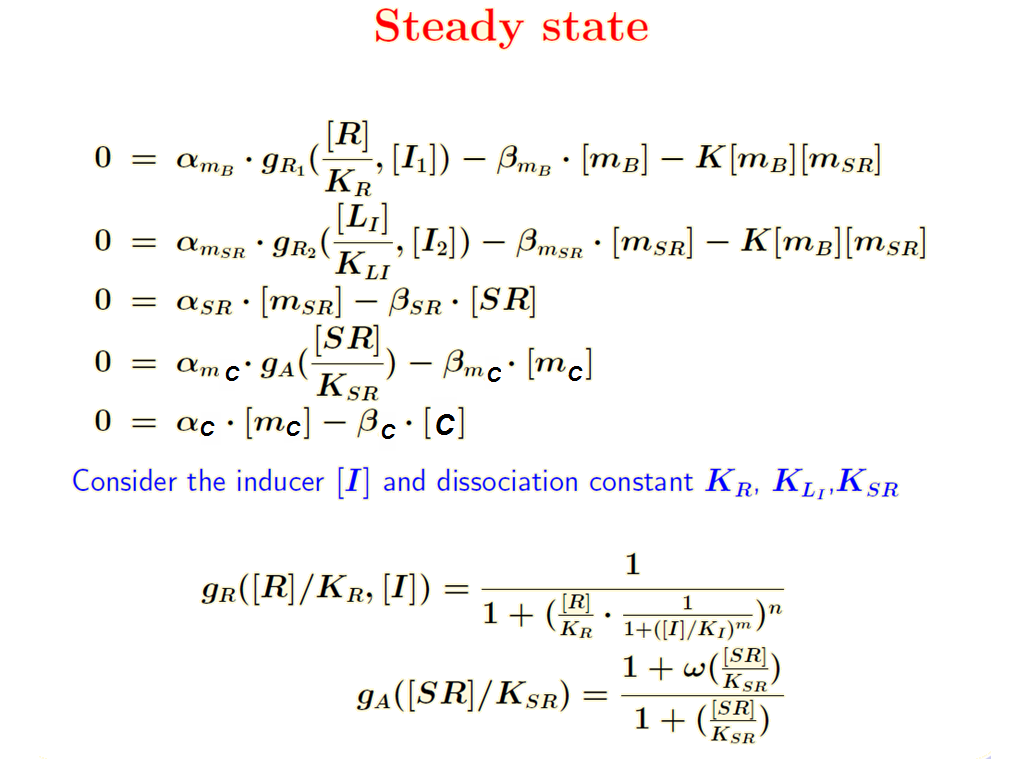Equation 3: The steady state analysis for sRNA-mediated regulation circuit.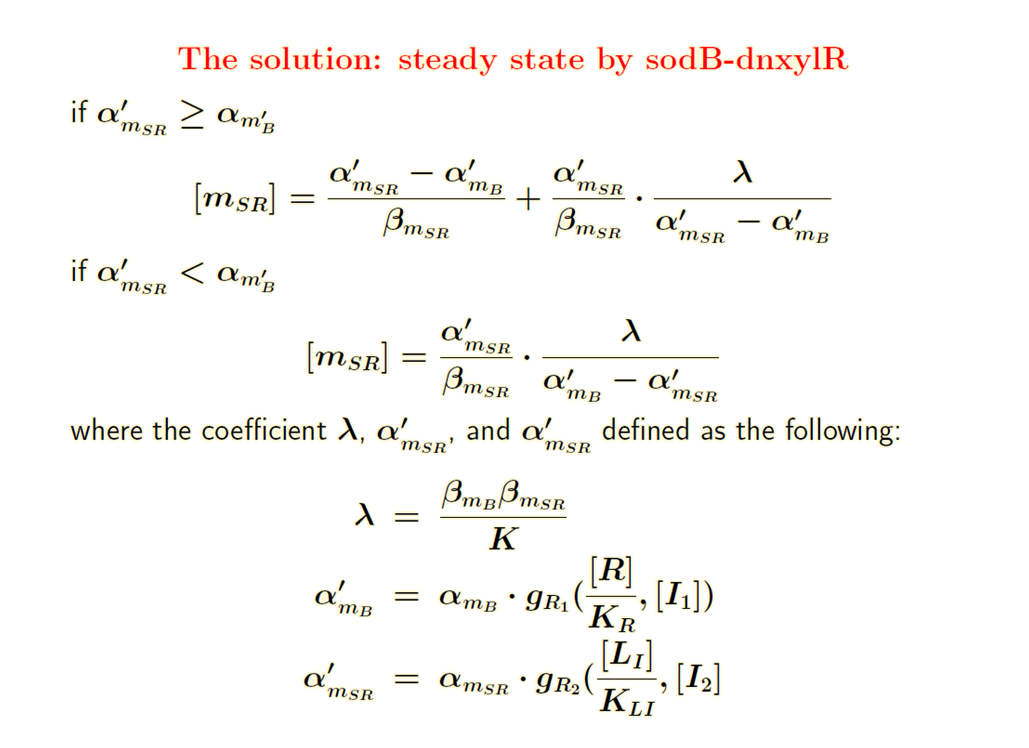Equation 4: The approximation approach based on the certain condition of transcription rateFigure 16.The sRNA-mediated regulation circuit with K = 0.02.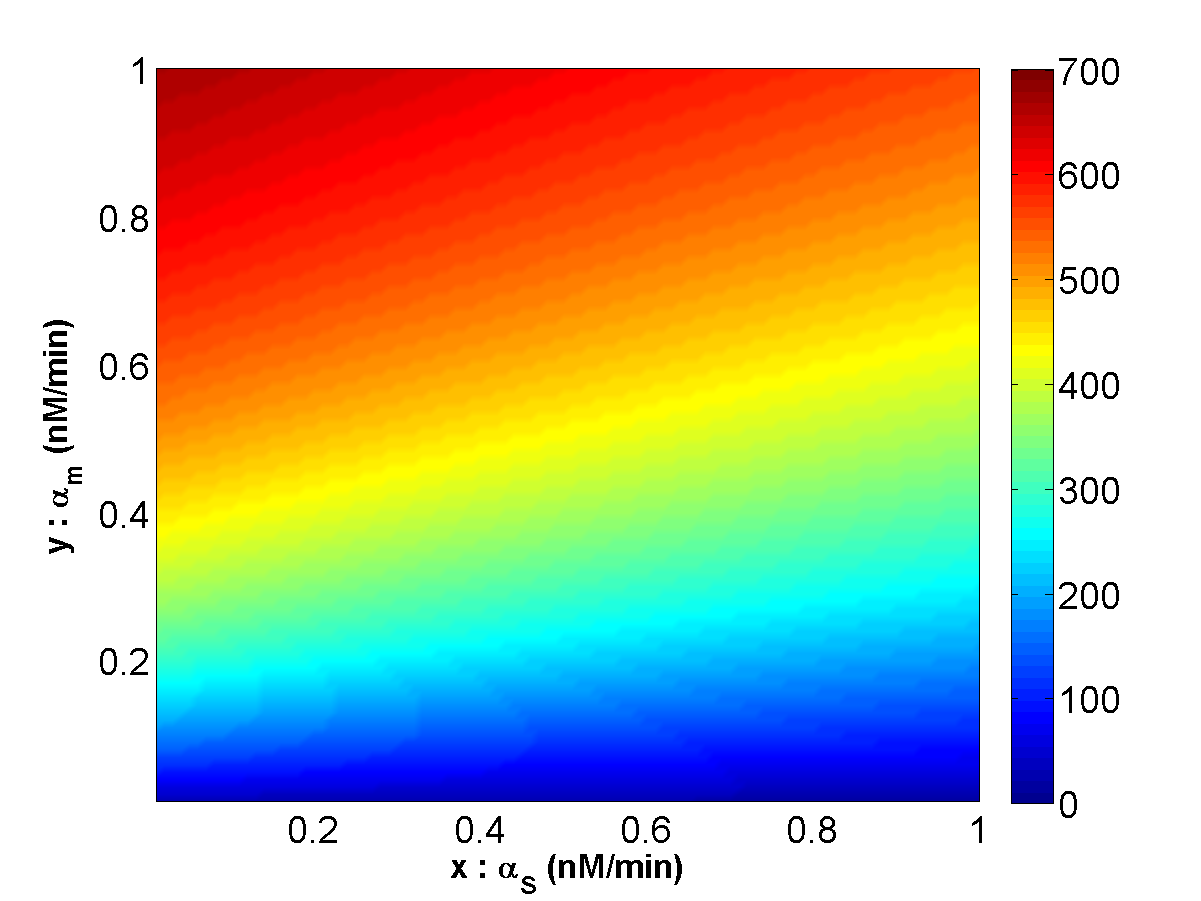Figure 17.The sRNA-mediated regulation circuit with K = 0.002.

Small regulatory RNA (sRNA) is responsible for coordination of gene expression network in both prokaryotes and eukaryotes. Some critical genetic regulation circuits in prokaryotes are mostly controlled by the binding of sRNA and the related target mRNA. In this section, we analyze the phase space of the synthetic regulatory circuit in Escherichia Coli by utilizing molecular dynamic simulations to calculate their related binding constants K. The computed results illustrate a chemostat that allows us to probe various aspects of the sRNA with their related targets. The analysis of the parameter phase regulated by the synthetic circuit also sheds light on possible functional roles of sRNA to indicate the regulatory feedback motifs controlling a large number of bio-synthetic operons in bacteria.

Code 1 (C++): Multi_gR.cpp

//////////////multi_gR.cpp//////////////////////////////////////////////////
/*
Info   :  multi_g_R.cpp is the code with which you can calculate the gR function of reporter-inducer
for various [Inducer].
Author : Eddie Wang  eddiewang0902@gmail.com
Date   : 20161019
Usage  : There are two systems that you can calcaulte for K_plac-LacI = 550 nM (0.55 uM),
K_lacI-IPTG = 30000 nM(30 uM) and K_pTet-TetR = 50 nM (0.05 uM), K_TetR-aTc = 15 nM (0.015 uM),
i represents the [inducer]. R is [protein]. You can plot the output data by Matlab or others.
*/
#include <iostream>
#include <system.hpp>
#include <string>
#include <stdio.h>
#include <stdlib.h>
#include <conio.h>
#include <math.h>

#define hmt 20000// how many times

using namespace std;
double g_R(double R, double K_R, double K_I, double I, int m, int n);

int main()
{
FILE *pFile;

//    IPTG and PlacI use uM
//    double R = 10.0;
//    Tc and Ptet use  nM
double R = 1000.0;

AnsiString Path_str = "D:\\research5_PHLee\\sodB_ryhB\\code\\multi_g_R\\m_file_plot\\multi_g_R_SmP\\";
for (int j = 1; j <= 5; j++)
{
AnsiString File_str = "multi_g_R_SmP_";
File_str += j;
File_str += ".txt";
AnsiString Path = Path_str + File_str;
pFile = fopen ( Path.c_str(),"w");
double ratio;

// K_plac-LacI = 550 nM (0.55 uM), K_lacI-IPTG = 30000 nM(30 uM)
// K_pTet-TetR = 50 nM (0.05 uM), K_TetR-aTc = 15 nM (0.015 uM)
// n_plac =2, 3  , n_pTet = 3.0  cooperativity number

// PlacI
//      double K_R = 0.55; // uM
//      double K_I = 30  ;  // uM

// PtetR
double K_R = 15; // nM
double K_I = 50  ;  // nM
// -- LacI  i = 400
//---TetR   i = 1000

int m = 2;
int n = 3;
for (int i = 0; i <= 1000; i++) {
ratio = g_R(R, K_R, K_I, i,m,n);
fprintf(pFile,"%3d , %10f, %10f, %10f, %10f, %5d, %5d\n", i, ratio, R, K_R, K_I, m, n);
}
fclose(pFile);
//   LacI  R= 10
//      R -= 2.0;

//   Ptet  R= 1000
R -= 200.0;
}

return 0;
}

double g_R(double R, double K_R, double K_I, double I, int m, int n)
{
double x = R / K_R;
double temp = x * 1.0 / (1.0 + pow(I / K_I, m));
return 1.0 / (1.0 + pow(temp, n));
}

//////////////multi_gR.cpp////////////////////////////////////////////////



Code 2 (matlab): Eddie_plot_gR_PtetR_aTc.m

%%%%%%%%%%%%%% Eddie_plot_gR_PtetR_aTc.m %%%%%%%%%%%%%%%%%%%%%%%%%%%%%%%%%%%%%%%%%%%%%%%%%%%%%%%
%Info   :  Eddie_plot_gR_PtetR_aTc.m is designed to read the output from multi_g_R.cpp,
%          meaning that you can calculate the gR function first by multi_g_R.cpp and then plot
%          gR for [reporter] vs. [inducer]. There are two systems that you can calculate,
%          (1) K_plac-LacI = 550 nM (0.55 uM), K_lacI-IPTG = 30000 nM(30 uM) and (2)
%          K_pTet-TetR = 50 nM (0.05 uM), K_TetR-aTc = 15 nM (0.015 uM). i represents
%          the [inducer] and R is [protein]. You can choose what kind of protein you want to plot.
%Author : Eddie Wang  eddiewang0902@gmail.com
%Date   : 20161019
%Usage  : (1) prepare the proper folder
%         (2) Eddie_plot_gR_PtetR_aTc('multi_g_R_SmP');
%%%%%%%%%%%%%%%%%%%%%%%%%%%%%%%%%%%%%%%%%%%%%%%%%%%%%%%%%%%%%%%%%%%%%%%%%%%%%%%%%%%%%%%%%%%%%%%%

function b = Eddie_plot_gR_PtetR_aTc(file)

% Read data from the txt file
cstring='rgbcmky'; % color string
figure;
for j=1:6,
filename = [file, '_', num2str(j), '.txt'];
foldname = [file '\'];
path = ['D:\Eddie\sodB_ryhB\code\multi_g_R\m_file_plot\' foldname filename];
if  j == 6
plot(normal(:,1),normal(:,2),'--b','LineWidth',2);
else
plot(normal(:,1),normal(:,2) ,cstring(j),'LineWidth',2);
hold on;
end
%hold on;
end
set(gca, 'XTick',[ 0: 100 : 1000]);
set(gca, 'YTick',[ 0: 0.1 : 1]);
% PlacI and IPTG use uM, Ptet and TetR use nM
xlabel('[Tc] (nM)','FontSize',14,'FontWeight','bold');
%xlabel('Population','FontSize',12,'FontWeight','bold','Color','r')
%xlabel('[IPTG] (\muM)','FontSize',14,'FontWeight','bold');
ylabel('g_{R}','FontSize',14,'FontWeight','bold');
%legend('[LacI]: 10 \muM','[LacI]: 8 \muM','[LacI]: 6 \muM','[LacI]: 4 \muM','[LacI]: 2 \muM', 4);
%legend('[LacI]: 1 \muM','[LacI]: 0.8 \muM','[LacI]: 0.6 \muM','[LacI]: 0.4 \muM','[LacI]: 0.2 \muM', 4);
%legend('[TetR]: 0.1 \muM','[TetR]: 0.08 \muM','[TetR]: 0.06 \muM','[TetR]: 0.04 \muM','[TetR]: 0.02 \muM', 4);
legend('[TetR]: 1 \muM','[TetR]: 0.8 \muM','[TetR]: 0.6 \muM','[TetR]: 0.4 \muM','[TetR]: 0.2 \muM', 4);
set(gca,'FontSize',18);
filename = [file];
foldname = [file '\'];
path = ['D:\Eddie\sodB_ryhB\code\multi_g_R\m_file_plot\' foldname filename];
saveas(gcf,[path 'g_R.bmp'],'bmp');
saveas(gcf,[path 'g_R.png'],'png');
saveas(gcf,[path 'g_R.jpg'],'jpg');
saveas(gcf,[path 'g_R.eps'],'eps');

%%%%%%%%%%%%%% Eddie_plot_gR_PtetR_aTc.m %%%%%%%%%%%%%%%%%%%%%%%%%%%%%%%%%%%%%%%%%%%%%%%%%%%%%%%


Code 3 (C++): Cyp_sRNA.cpp

//////////////Cyp_sRNA.cpp//////////////////////////////////////////////////////////////////////////
/*
Info   :  Cyp_sRNA.cpp is the code with which you can calculate the sRNA-mediated genetic circuit
for various [Inducer].
Author : Eddie Wang  eddiewang0902@gmail.com
Date   : 20161019
Usage  : compile Cyp_sRNA.cpp and run the code to output data. You can plot the output data by Matlab.
*/
#include <stdio.h>
#include <stdlib.h>
#include <conio.h>
#include <math.h>

#define hmt 20000// After the time limit, you are able to get the output data.

void parasite(double t,double S,double m,double P, double m_z, double *dSdt,
double *dmdt, double *dPdt, double *dm_zdt, double a_S, double a_m, double k)
{
const double a_P = 0.1; // generation rate of Protein
//const double a_m = 0.2; // Transcription rate of target mRNA
//const double a_S = 0.1; // Transcription rate of small RNA
//const double k = 0.02;   // Binding rate of small RNA:mRNA complex

const double B_P = 0.01; // degradation rate of Protein
const double B_m = 0.1; // degradation rate of free mRNA
const double B_S = 0.02; // degradation rate of free small RNA
const double n_S = 1.0;    // Hill coefficient
const double n_P = 1.0;    // Hill coefficient
//  consider regulation gR =1
const double g_R1 = 1.0;  //g_s
const double g_R2 = 1.0;  //g_m

const double a_m_z = 0.1;
const double B_m_z = 0.01;
const double k_SR = 50.0;
const double w = 100.0;
double g_A = (1 + w * P / k_SR) / (1 + P / k_SR); // activation function

*dSdt= a_S * g_R1 - B_S * S - k * m * S;
*dmdt = a_m * g_R2 - B_m * m - k * m * S;
*dPdt = a_P * m - B_P * P;
*dm_zdt = a_m_z * g_A - B_m_z * m_z;

}

// Runge-Kutta 4 order method
void r_k(double h,double t, double *S, double *m,double *P,double *m_z,
double a_S, double a_m, double k)
{
double kS,km,kP,km_z;//runge kutta
//double dSdt,dmdt,dPdt,dm_zdt;
double rS,rm,rP,rm_z;

//compute k_1, store in rS, rm, rP
parasite(t, *S, *m, *P, *m_z, rS, rm, rP, rm_z, a_S, a_m, k);

//compute k_2, store in rs, rm
kS = *S + 0.5 * h * rS;
km = *m + 0.5 * h * rm;
kP = *P + 0.5 * h * rP;
km_z = *m_z + 0.5 * h * rm_z;
parasite(t + 0.5 * h, kS, km, kP, km_z, rS + 1, rm + 1, rP + 1, rm_z + 1, a_S, a_m, k);

//compute k_3, store in rs, rm
kS = *S + 0.5 * h * rS;
km = *m + 0.5 * h * rm;
kP = *P + 0.5 * h * rP;
km_z = *m_z + 0.5 * h * rm_z;
parasite(t + 0.5 * h, kS, km, kP, km_z, rS + 2, rm + 2, rP + 2, rm_z + 2, a_S, a_m, k);

//compute k_4, store in rs, rm
kS = *S + h * rS;
km = *m + h * rm;
kP = *P + h * rP;
km_z = *m_z + h * rm_z;
parasite(t + h, kS, km, kP, km_z, rS + 3, rm + 3, rP + 3, rm_z + 3, a_S, a_m, k);

//the Runge-Kutta method: compute next value
*S = *S + h * (rS + 2 * rS + 2 * rS + rS) / 6.0;
*m = *m + h * (rm + 2 * rm + 2 * rm + rm) / 6.0;
*P = *P + h * (rP + 2 * rP + 2 * rP + rP) / 6.0;
*m_z = *m_z + h * (rm_z + 2 * rm_z + 2 * rm_z + rm_z) / 6.0;
}

int main()
{
double h = 0.05;   // time
FILE *pFile;
pFile = fopen ("Saturation_SmP.txt","w");

double vS=0.05;      // sRNA
double vm=0.05;      // mRNA
double vP=0.05;      // Protein
double vm_z = 0.05; //  LacZ mRNA
double a_S = 0.0;   //  Transcription rate of small RNA
double a_m = 0.0;   //  Transcription rate of target mRNA
double k = 0.002;   //  binding constant K for RyhB-sodB
double stepSize = 0.01;
int steps = 0;
for (int i = 0; i < 100; i++)
{
a_S += stepSize;
for(int j = 0; j < 100; j++)
{
a_m += stepSize;
for(int counter = 0; counter < hmt; counter++)
{
r_k(h, h, &vS, &vm, &vP, &vm_z, a_S, a_m, k);
}
fprintf(pFile, "%6d  %6.4f %6.4f %10.6f  %10.6f  %10.6f\n", steps, a_S, a_m, vm, vp, vm_z);
steps++;
vS = 0.05;
vm = 0.05;
vP = 0.05;
vm_z = 0.05;
}
a_m = 0.0;
}
fclose(pFile);
return 0;
}
//////////////Cyp_sRNA.cpp//////////////////////////////////////////////////////////////////////////


Code 4 (matlab): Eddie_plot_mesh_Cyp_sRNA.m

%%%%%%%%%%%%%% Eddie_plot_mesh_Cyp_sRNA.m %%%%%%%%%%%%%%%%%%%%%%%%%%%%%%%%%%%%%%%%%%%%%%%%%%%%%%%
%Info   :  Eddie_plot_mesh_Cyp_sRNA.m is designed to read the output from Cyp_sRNA.cpp,
%          meaning that you can calculate the rate equation first and then plot
%          phase space with various a_S and a_m in 2-D and 3-D mode. You have to define the saturation
%          state by yourself for caxis([0 10].
%Author : Eddie Wang  eddiewang0902@gmail.com
%Date   : 20161019
%Usage  : (1) prepare the proper folder
%         (2) Eddie_plot_mesh_Cyp_sRNA('Saturation_SmP');
%%%%%%%%%%%%%%%%%%%%%%%%%%%%%%%%%%%%%%%%%%%%%%%%%%%%%%%%%%%%%%%%%%%%%%%%%%%%%%%%%%%%%%%%%%%%%%%%
function b = Eddie_plot_mesh_Cyp_sRNA(file)

% Read data from the txt file
filename = [file '.txt'];
foldname = [file '\'];
path = ['D:\Eddie\parasite_small_RNA\code\Satruation\m_file_plot\' foldname filename];

figure;
x = normal(:,2);
y = normal(:,3);
z = normal(:,4);
dim = sqrt(size(x));
dim = dim(1);
xx = reshape(x, dim, dim);
yy = reshape(y, dim, dim);
zz = reshape(z, dim, dim);
pcolor(xx, yy, zz);
caxis([0 10]);
colorbar;
colormap('default');
xlabel('x : \alpha_S (nM/min)','FontSize',14,'FontWeight','bold');
ylabel('y : \alpha_m (nM/min)','FontSize',14,'FontWeight','bold');
saveas(gcf,[path '_sRNA_as_am_2D.jpg'],'jpg');
saveas(gcf,[path '_sRNA_as_am_2D.png'],'png');
saveas(gcf,[path '_sRNA_as_am_2D.eps'],'eps');

figure;
surf(xx, yy, zz);
caxis([0 10]);
colorbar;
colormap('default');
xlabel('x: \alpha_S','FontSize',14,'FontWeight','bold');
ylabel('y: \alpha_m','FontSize',14,'FontWeight','bold');
zlabel('target mRNA concentration(nM)');
saveas(gcf,[path '_sRNA_as_am_3D.jpg'],'jpg');
saveas(gcf,[path '_sRNA_as_am_3D.png'],'png');
saveas(gcf,[path '_sRNA_as_am_3D.eps'],'eps');
%%%%%%%%%%%%%% Eddie_plot_mesh_Cyp_sRNA.m %%%%%%%%%%%%%%%%%%%%%%%%%%%%%%%%%%%%%%%%%%%%%%%%%%%%%%%


Reference

[ 1] Cheng-Ping Jheng, Shih-Wei Wang, Kuan-Ling Chen, Tzu-Han Chen, Shang-Yu Chou, Wan-Sheng Su, Po-Han Lee, Cheng-I Lee, The Computational Determination of Small RNA Binding Constant to Clarify the Synthetic Regulatory Circuit in Escherichia coli, Biophysical Journal 110(3), Supplement 1 (2016) p315a–316a

[ 2] Keseler, I. M. et al., EcoCyc: fusing model organism databases with systems biology. Nucleic acids research 41 (2013) D605-612.

[ 3] Altschul, S. F., W. Gish, W. Miller, E. W. Myers, and D. J. Lipman, Basic local alignment search tool. Journal of molecular biology 215 (1990) 403-410.

[ 4] Madden, T. L., R. L. Tatusov, and J. Zhang., Applications of network BLAST server. Methods in enzymology 266 (1996) 131-141.

[ 5] Zhang, Z., S. Schwartz, L. Wagner, and W. Miller, A greedy algorithm for aligning DNA sequences. Journal of computational biology, Journal of computational molecular cell biology 7 (2000) 203-214.

[ 6] Anandakrishnan, R., B. Aguilar, and A. V. Onufriev, H++ 3.0: automating pK prediction and the preparation of biomolecular structures for atomistic molecular modeling and simulations. Nucleic acids research 40 (2012) W537-541.

[ 7] Myers, J., G. Grothaus, S. Narayanan, and A. Onufriev, A simple clustering algorithm can be accurate enough for use in calculations of pKs in macromolecules. Proteins 63 (2006) 928-938.

[ 8] Gordon, J. C., J. B. Myers, T. Folta, V. Shoja, L. S. Heath, and A. Onufriev, H++: a server for estimating pKas and adding missing hydrogens to macromolecules. Nucleic acids research 33 (2005) W368-371.

[ 9] Case, D., J. Berryman, R. Betz, D. Cerutti, T. Cheatham III, T. Darden, R. Duke, T. Giese, H. Gohlke, and A. Goetz. AMBER 2015, University of California, San Francisco.

 Maier, J. A., C. Martinez, K. Kasavajhala, L. Wickstrom, K. E. Hauser, and C. Simmerling, ff14SB: Improving the Accuracy of Protein Side Chain and Backbone Parameters from ff99SB. Journal of chemical theory and computation 11 (2015) 3696-3713.

 Salomon-Ferrer, R., A. W. Gotz, D. Poole, S. Le Grand, and R. C. Walker, Routine Microsecond Molecular Dynamics Simulations with AMBER on GPUs. 2. Explicit Solvent Particle Mesh Ewald. Journal of chemical theory and computation 9 (2013) 3878-3888.

 Gotz, A. W., M. J. Williamson, D. Xu, D. Poole, S. Le Grand, and R. C. Walker, Routine Microsecond Molecular Dynamics Simulations with AMBER on GPUs. 1. Generalized Born. Journal of chemical theory and computation 8 (2012) 1542-1555.

 Le Grand, S., A. W. Götz, and R. C. Walker, SPFP: Speed without compromise—A mixed precision model for GPU accelerated molecular dynamics simulations. Computer Physics Communications 184 (2013) 374-380.

 Tsui, V., and D. A. Case, Theory and applications of the generalized Born solvation model in macromolecular simulations. Biopolymers 56 (2000) 275-291.

 Genheden, S., and U. Ryde, The MM/PBSA and MM/GBSA methods to estimate ligand-binding affinities. Expert opinion on drug discovery 10 (2015) 449-461.

 Weis, A., K. Katebzadeh, P. Soderhjelm, I. Nilsson, and U. Ryde, Ligand affinities predicted with the MM/PBSA method: dependence on the simulation method and the force field. Journal of medicinal chemistry 49 (2006) 6596-6606.

 Xu, L., H. Sun, Y. Li, J. Wang, and T. Hou, Assessing the performance of MM/PBSA and MM/GBSA methods. 3. The impact of force fields and ligand charge models. The journal of physical chemistry. B 117 (2013) 8408-8421.

 Nellen, Wolfgang, Hammann, Christian, Small RNAs: Analysis and Regulatory Functions. Springer-Verlag Berlin Heidelberg (2006).

 Tafer, H., F. Amman, F. Eggenhofer, P. F. Stadler, and I. L. Hofacker, Fast accessibility-based prediction of RNA-RNA interactions. Bioinformatics (Oxford, England) 27 (2011) 1934-1940.

 E. Levine, Z. Zhang, T. Kuhlman, T. Hwa, Quantitative Characteristics of Gene Regulation Mediated by small RNA, PLoS Biol 5 (2007) 1998-2010.